# Lane Detection in 3-D Lidar Point Cloud

This example shows how to detect lanes in lidar point clouds. You can use the intensity values returned from lidar point clouds to detect ego vehicle lanes. You can further improve the lane detection by using a curve-fitting algorithm and tracking the curve parameters. Lidar lane detection enables you to build complex workflows like lane keep assist, lane departure warning, and adaptive cruise control for autonomous driving. A test vehicle collects the lidar data using a lidar sensor mounted on its rooftop.

### Introduction

Lane detection in lidar involves detection of the immediate left and right lanes, also known as ego vehicle lanes, with respect to the lidar sensor. It involves the following steps:

• Region of interest extraction

• Ground plane segmentation

• Peak intensity detection

• Lane detection using window search

• Parabolic polynomial fitting

• Parallel lane fitting

• Lane tracking

This flowchart gives an overview of the workflow presented in this example.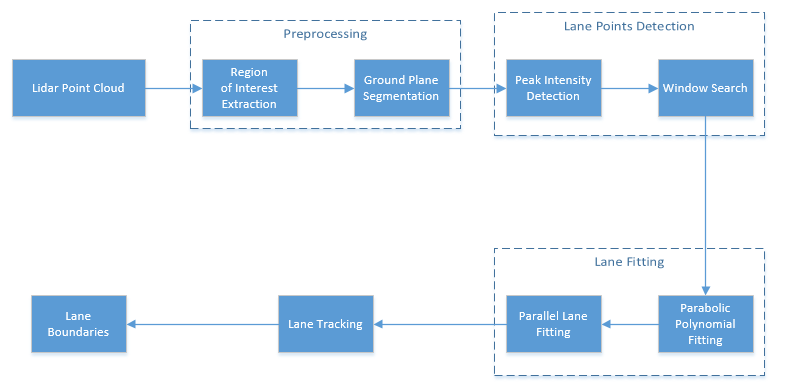The advantages of using lidar data for lane detection are :

• Lidar point clouds give a better 3-D representation of the road surface than image data, thus reducing the required calibration parameters to find the bird's-eye view.

• Lidar is more robust against adverse climatic conditions than image-based detection.

• Lidar data has a centimeter level of accuracy, leading to accurate lane localization.

The lidar data used in this example has been collected using the Ouster OS1-64 channel lidar sensor, producing high-resolution point clouds. This data set contains point clouds stored as a cell array of `pointCloud` object. Each 3-D point cloud consists of XYZ locations along with intensity information.

Note: Download time of the data depends on your internet connection. MATLAB will be temporarily unresponsive during the execution of this code block.

```% Download lidar data lidarData = helperGetDataset;```

Selecting the first frame of the dataset for further processing.

```% Select first frame ptCloud = lidarData{1}; % Visualize input point cloud figure pcshow(ptCloud) title('Input Lidar Point Cloud') axis([0 50 -15 15 -5 5]) view([-42 35])```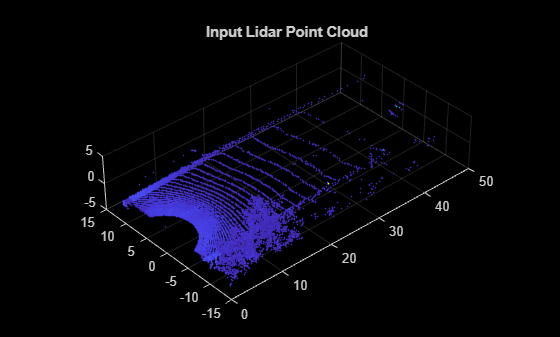### Preprocessing

To estimate the lane points, first preprocess the lidar point clouds. Preprocessing involves the following steps:

• Region of interest extraction

• Ground plane segmentation

```% Define ROI in meters xlim = [5 55]; ylim = [-3 3]; zlim = [-4 1]; roi = [xlim ylim zlim]; % Crop point cloud using ROI indices = findPointsInROI(ptCloud,roi); croppedPtCloud = select(ptCloud,indices); % Remove ground plane maxDistance = 0.1; referenceVector = [0 0 1]; [model,inliers,outliers] = pcfitplane(croppedPtCloud,maxDistance,referenceVector); groundPts = select(croppedPtCloud,inliers); figure pcshow(groundPts) title('Ground Plane') view(3)```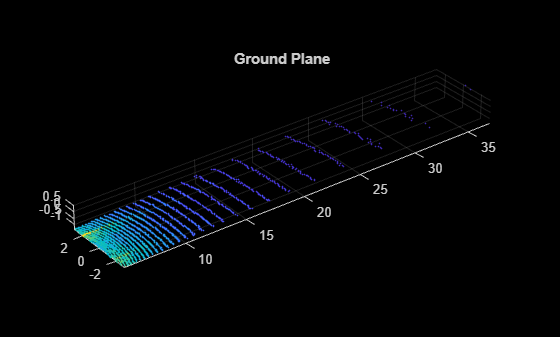### Lane Point Detection

Detect lane points by using a sliding window search, where the initial estimates for the sliding windows are made using an intensity-based histogram.

Lane point detection consists primarily of these two steps:

• Peak intensity detection

• Window search

#### Peak Intensity Detection

Lane points in the lidar point cloud have a distinct distribution of intensities. Usually, these intensities occupy the upper region in the histogram distribution and appear as high peaks. Compute a histogram of intensities from the detected ground plane along the ego vehicle axis (positive X-axis). The `helperComputeHistogram` helper function creates a histogram of intensity points. Control the number of bins for the histogram by specifying the bin resolution.

```histBinResolution = 0.2; [histVal,yvals] = helperComputeHistogram(groundPts,histBinResolution); figure plot(yvals,histVal,'--k') set(gca,'XDir','reverse') hold on```

Obtain peaks in the histogram by using the `helperfindpeaks` helper function. Further filter the possible lane points based on lane width using the `helperInitialWindow` helper function.

```[peaks,locs] = helperfindpeaks(histVal); startYs = yvals(locs); laneWidth = 4; [startLanePoints,detectedPeaks] = helperInitialWindow(startYs,peaks,laneWidth); plot(startYs,peaks,'*r') plot(startLanePoints,detectedPeaks,'og') legend('Histogram','Peak','Detected Peaks','Location','North') title('Peak Detection') hold off```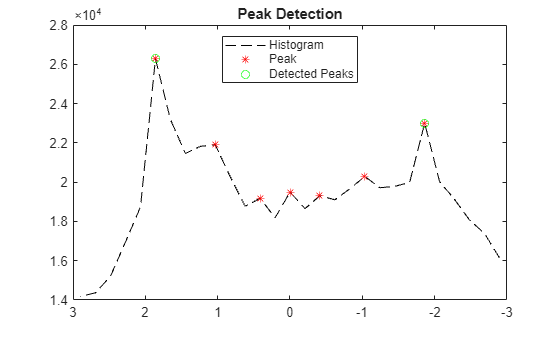#### Window Search

Window search is used to detect lane points by sliding the windows along the lane curvature direction. Window search consists of two steps:

• Window initialization

• Sliding window

#### Window Initialization

The detected peaks help in the initialization of the search window. Initialize the search windows as multiple bins with red and blue colors for left and right lanes respectively.

```vBinRes = 1; hBinRes = 0.8; numVerticalBins = ceil((groundPts.XLimits(2) - groundPts.XLimits(1))/vBinRes); laneStartX = linspace(groundPts.XLimits(1),groundPts.XLimits(2),numVerticalBins); % Display bin windows figure pcshow(groundPts) view(2) helperDisplayBins(laneStartX,startLanePoints(1),hBinRes/2,groundPts,'red'); helperDisplayBins(laneStartX,startLanePoints(2),hBinRes/2,groundPts,'blue'); title('Initialized Sliding Windows')```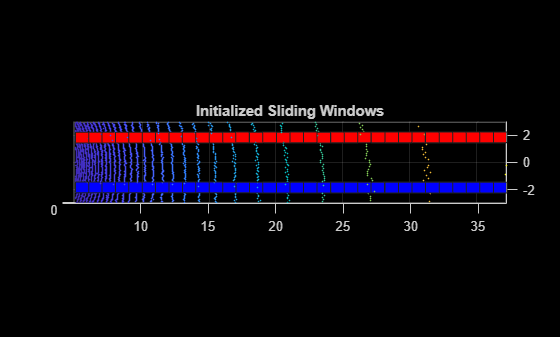#### Sliding Window

The sliding bins are initialized from the bin locations, and iteratively move along the ego vehicle (positive X-axis) direction. The `helperDetectLanes` helper function detects lane points and visualizes the sliding bins. Consecutive bins slide along the Y-axis based upon the intensity values inside the previous bin.

At regions where the lane points are missing, the function predicts the sliding bins using second-degree polynomial. This condition commonly arises when there are moving object's crossing the lanes. The sliding bins are yellow and the bins that are predicted using the polynomial are green.

```display = true; lanes = helperDetectLanes(groundPts,hBinRes, ... numVerticalBins,startLanePoints,display);```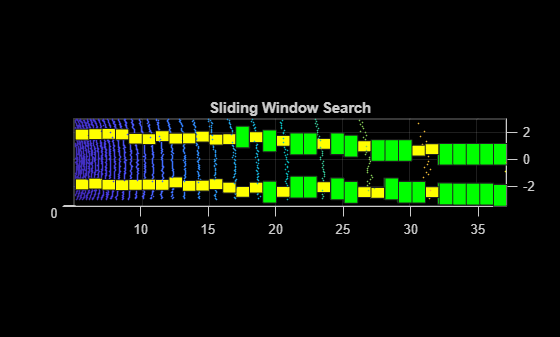```% Plot final lane points lane1 = lanes{1}; lane2 = lanes{2}; figure pcshow(groundPts) title('Detected Lane Points') hold on p1 = plot3(lane1(:,1),lane1(:,2),lane1(:,3),'*y'); p2 = plot3(lane2(:,1),lane2(:,2),lane2(:,3),'*r'); hold off view(2) lgnd = legend([p1 p2],{'Left Lane Points','Right Lane Points'}); set(lgnd,'color','White','Location','southoutside')```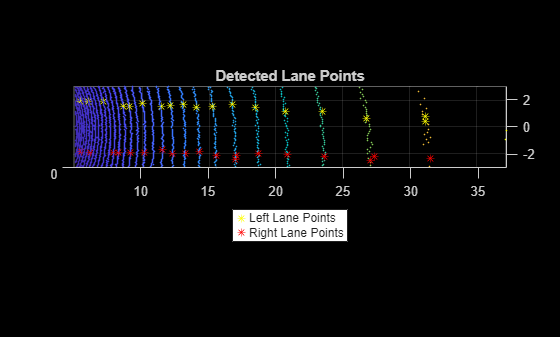### Lane Fitting

Lane fitting involves estimating a polynomial curve on the detected lane points. These polynomials are used along with parallel lane constraint for lane fitting.

#### Parabolic Polynomial Fitting

The polynomial is fitted on X-Y points using a 2-degree polynomial represented as $\mathit{a}{\mathit{x}}^{2}+\mathit{bx}+\mathit{c}$, where a, b, and c are polynomial parameters. To perform curve fitting, use the `helperFitPolynomial` helper function, which also handles outliers using the random sample consensus (RANSAC) algorithm. To estimate the 3-D lane points, extract the parameters from the plane model created during the preprocessing step. The plane model is represented as $\mathit{ax}+\mathit{by}+\mathit{cz}+\mathit{d}=0$, where the Z-coordinate is unknown. Estimate the Z-coordinate by substituting the X- and Y-coordinates in the plane equation.

```[P1,error1] = helperFitPolynomial(lane1(:,1:2),2,0.1); [P2,error2] = helperFitPolynomial(lane2(:,1:2),2,0.1); xval = linspace(5,40,80); yval1 = polyval(P1,xval); yval2 = polyval(P2,xval); % Z-coordinate estimation modelParams = model.Parameters; zWorld1 = (-modelParams(1)*xval - modelParams(2)*yval1 - modelParams(4))/modelParams(3); zWorld2 = (-modelParams(1)*xval - modelParams(2)*yval2 - modelParams(4))/modelParams(3); % Visualize fitted lane figure pcshow(croppedPtCloud) title('Fitted Lane Polynomial') hold on p1 = plot3(xval,yval1,zWorld1,'y','LineWidth',0.2); p2 = plot3(xval,yval2,zWorld2,'r','LineWidth',0.2); lgnd = legend([p1 p2],{'Left Lane Points','Right Lane Points'}); set(lgnd,'color','White','Location','southoutside') view(2) hold off```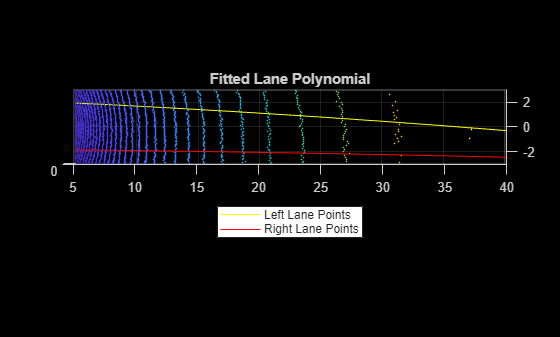#### Parallel Lane Fitting

The lanes are usually parallel to each other along the road. To make the lane fitting robust, use this parallel constraint. When fitting the polynomials, the `helperFitPolynomial` helper function also computes, the fitting error. Update the lanes having erroneous points with the new polynomial. Update this polynomial by shifting it along the Y-axis.

```lane3d1 = [xval' yval1' zWorld1']; lane3d2 = [xval' yval2' zWorld2']; % Shift the polynomial with a high score along the Y-axis towards % the polynomial with a low score if error1 > error2 lanePolynomial = P2; if lane3d1(1,2) > 0 lanePolynomial(3) = lane3d2(1,2) + laneWidth; else lanePolynomial(3) = lane3d2(1,2) - laneWidth; end lane3d1(:,2) = polyval(lanePolynomial,lane3d1(:,1)); lanePolynomials = [lanePolynomial; P2]; else lanePolynomial = P1; if lane3d2(1,2) > 0 lanePolynomial(3) = lane3d1(1,2) + laneWidth; else lanePolynomial(3) = lane3d1(1,2) - laneWidth; end lane3d2(:,2) = polyval(lanePolynomial,lane3d2(:,1)); lanePolynomials = [P1; lanePolynomial] end % Visualize lanes after parallel fitting figure pcshow(ptCloud) axis([0 50 -15 15 -5 5]) hold on p1 = plot3(lane3d1(:,1),lane3d1(:,2),lane3d1(:,3),'y','LineWidth',0.2); p2 = plot3(lane3d2(:,1),lane3d2(:,2),lane3d2(:,3),'r','LineWidth',0.2); view([-90 90]) title('Fitted Lanes') lgnd = legend([p1 p2],{'Left Lane Points','Right Lane Points'}); set(lgnd,'color','White','Location','southoutside') hold off```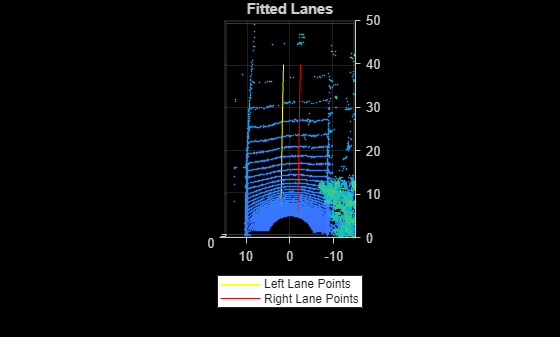### Lane Tracking

Lane tracking helps in stabilizing the lane curvature caused by sudden jerks and drifts. These changes can occur because of missing lane points, vehicles moving over the lanes, and erroneous lane detection. Lane tracking is a two-step process:

• Track lane polynomial parameters$\left(\mathit{a},\text{\hspace{0.17em}}\mathit{b}\right)$to control the curvature of the polynomial.

• Track the start points coming from peak detection. This parameter is denoted as $\mathit{c}$ in the polynomial.

These parameters are updated using a Kalman filter with a constant acceleration motion model. To initiate a Kalman filter, use the configureKalmanFilter.

```% Initial values curveInitialParameters = lanePolynomials(1,1:2); driftInitialParameters = lanePolynomials(:,3)'; initialEstimateError = [1 1 1]*1e-1; motionNoise = [1 1 1]*1e-7; measurementNoise = 10; % Configure Kalman filter curveFilter = configureKalmanFilter('ConstantAcceleration', ... curveInitialParameters,initialEstimateError,motionNoise,measurementNoise); driftFilter = configureKalmanFilter('ConstantAcceleration', ... driftInitialParameters,initialEstimateError,motionNoise,measurementNoise);```

### Loop Through Data

Loop through and process the lidar data by using the `helperLaneDetector` helper class. This helper class implements all previous steps, and also performs additional preprocessing to remove the vehicles from the point cloud. This ensures that the detected ground points are flat and the plane model is accurate. The class method `detectLanes` detects and extracts the lane points for the left and right lane as a two-element cell array, where the first element corresponds to the left lane and the second element to the right lane.

```% Initialize the random number generator rng(2020) numFrames = numel(lidarData); detector = helperLaneDetector('ROI',[5 40 -3 3 -4 1]); % Turn on display player = pcplayer([0 50],[-15 15],[-5 5]); drift = zeros(numFrames,1); filteredDrift = zeros(numFrames,1); curveSmoothness = zeros(numFrames,1); filteredCurveSmoothness = zeros(numFrames,1); for i = 1:numFrames ptCloud = lidarData{i}; % Detect lanes detectLanes(detector,ptCloud); % Predict polynomial from Kalman filter predict(curveFilter); predict(driftFilter); % Correct polynomial using Kalman filter lanePolynomials = detector.LanePolynomial; drift(i) = mean(lanePolynomials(:,3)); curveSmoothness(i) = mean(lanePolynomials(:,1)); updatedCurveParams = correct(curveFilter,lanePolynomials(1,1:2)); updatedDriftParams = correct(driftFilter,lanePolynomials(:,3)'); % Update lane polynomials updatedLanePolynomials = [repmat(updatedCurveParams,[2 1]),updatedDriftParams']; % Estimate new lane points with updated polynomial lanes = updateLanePolynomial(detector,updatedLanePolynomials); filteredDrift(i) = mean(updatedLanePolynomials(:,3)); filteredCurveSmoothness(i) = mean(updatedLanePolynomials(:,1)); % Visualize lanes after parallel fitting ptCloud.Color = uint8(repmat([0 0 255],ptCloud.Count,1)); lane3dPc1 = pointCloud(lanes{1}); lane3dPc1.Color = uint8(repmat([255 0 0],lane3dPc1.Count,1)); lanePc = pccat([ptCloud lane3dPc1]); lane3dPc2 = pointCloud(lanes{2}); lane3dPc2.Color = uint8(repmat([255 255 0],lane3dPc2.Count,1)); lanePc = pccat([lanePc lane3dPc2]); view(player,lanePc) end```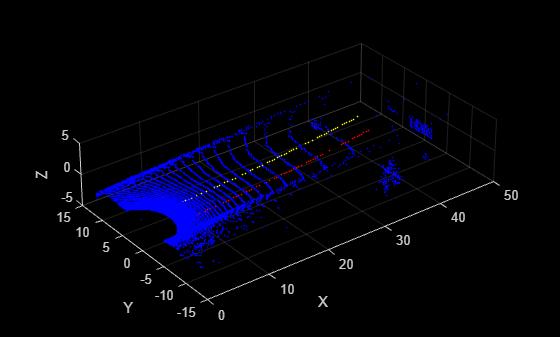### Results

To analyze the lane detection results, compare them against the tracked lane polynomials by plotting them in figures. Each plot compares the parameters with and without the Kalman filter. The first plot compares the drift of lanes along the Y-axis, and the second plot compares the smoothness of the lane polynomials. Smoothness is defined as the rate of change of the slope of the lane curve.

```figure plot(drift) hold on plot(filteredDrift) hold off title('Lane Drift Along Y-axis') legend('Drift Values','Filtered Drift Values')```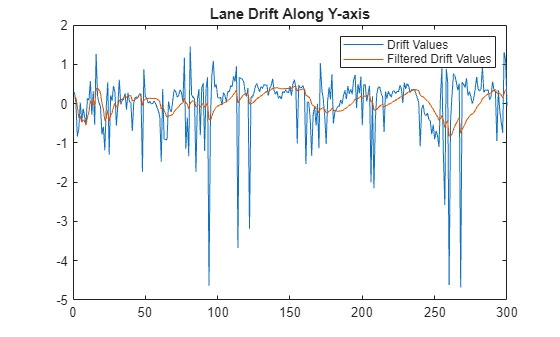```figure plot(curveSmoothness) hold on plot(filteredCurveSmoothness) hold off title('Curve Smoothness') legend('Curve Smoothness','Filtered Curve Smoothness')```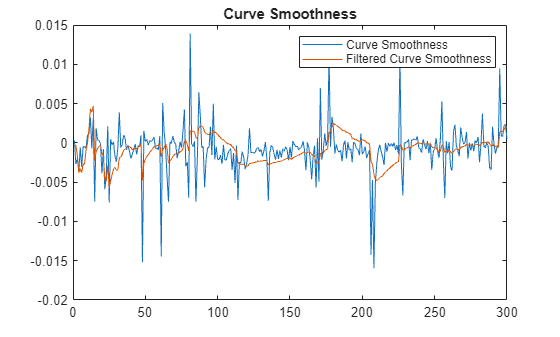### Summary

This example has shown you how to detect lanes on the intensity channel of point clouds coming from lidar sensor. You have also learned how to fit a 2-D polynomial on detected lane points, and leverage ground plane model to estimate 3-D lane points. You have also used Kalman filter tracking to further improve lane detection.

### Supporting Functions

The `helperLoadData` helper function loads the lidar data set into the MATLAB workspace.

```function reflidarData = helperGetDataset() lidarDataTarFile = matlab.internal.examples.downloadSupportFile( ... 'lidar','data/WPI_LidarData.tar.gz'); [outputFolder,~,~] = fileparts(lidarDataTarFile); % Check if tar.gz file is downloaded, but not uncompressed if ~exist(fullfile(outputFolder,'WPI_LidarData.mat'),'file') untar(lidarDataTarFile,outputFolder); end % Load lidar data load(fullfile(outputFolder,'WPI_LidarData.mat'),'lidarData'); % Select region with a prominent intensity value reflidarData = cell(300,1); count = 1; roi = [-50 50 -30 30 -inf inf]; for i = 81:380 pc = lidarData{i}; ind = findPointsInROI(pc,roi); reflidarData{count} = select(pc,ind); count = count+1; end end```

This `helperInitialWindow` helper function computes the starting points of the search window using the detected histogram peaks.

```function [yval,detectedPeaks] = helperInitialWindow(yvals,peaks,laneWidth) leftLanesIndices = yvals >= 0; rightLanesIndices = yvals < 0; leftLaneYs = yvals(leftLanesIndices); rightLaneYs = yvals(rightLanesIndices); peaksLeft = peaks(leftLanesIndices); peaksRight = peaks(rightLanesIndices); diff = zeros(sum(leftLanesIndices),sum(rightLanesIndices)); for i = 1:sum(leftLanesIndices) for j = 1:sum(rightLanesIndices) diff(i,j) = abs(laneWidth - (leftLaneYs(i) - rightLaneYs(j))); end end [~,minIndex] = min(diff(:)); [row,col] = ind2sub(size(diff),minIndex); yval = [leftLaneYs(row) rightLaneYs(col)]; detectedPeaks = [peaksLeft(row) peaksRight(col)]; estimatedLaneWidth = leftLaneYs(row) - rightLaneYs(col); % If the calculated lane width is not within the bounds, % return the lane with highest peak if abs(estimatedLaneWidth - laneWidth) > 0.5 if max(peaksLeft) > max(peaksRight) yval = [leftLaneYs(maxLeftInd) NaN]; detectedPeaks = [peaksLeft(maxLeftInd) NaN]; else yval = [NaN rightLaneYs(maxRightInd)]; detectedPeaks = [NaN rightLaneYs(maxRightInd)]; end end end```

This `helperFitPolynomial` helper function fits a RANSAC-based polynomial to the detected lane points and computes the fitting score.

```function [P,score] = helperFitPolynomial(pts,degree,resolution) P = fitPolynomialRANSAC(pts,degree,resolution); ptsSquare = (polyval(P,pts(:,1)) - pts(:,2)).^2; score = sqrt(mean(ptsSquare)); end```

This `helperComputeHistogram` helper function computes the histogram of the intensity values of the point clouds.

```function [histVal,yvals] = helperComputeHistogram(ptCloud,histogramBinResolution) numBins = ceil((ptCloud.YLimits(2) - ptCloud.YLimits(1))/histogramBinResolution); histVal = zeros(1,numBins-1); binStartY = linspace(ptCloud.YLimits(1),ptCloud.YLimits(2),numBins); yvals = zeros(1,numBins-1); for i = 1:numBins-1 roi = [-inf 15 binStartY(i) binStartY(i+1) -inf inf]; ind = findPointsInROI(ptCloud,roi); subPc = select(ptCloud,ind); if subPc.Count histVal(i) = sum(subPc.Intensity); yvals(i) = (binStartY(i) + binStartY(i+1))/2; end end end```

This `helperfindpeaks` helper function extracts peaks from the histogram values.

```function [pkHistVal,pkIdx] = helperfindpeaks(histVal) pkIdxTemp = (1:size(histVal,2))'; histValTemp = [NaN; histVal'; NaN]; tempIdx = (1:length(histValTemp)).'; % keep only the first of any adjacent pairs of equal values (including NaN) yFinite = ~isnan(histValTemp); iNeq = [1; 1 + find((histValTemp(1:end-1) ~= histValTemp(2:end)) & ... (yFinite(1:end-1) | yFinite(2:end)))]; tempIdx = tempIdx(iNeq); % Take the sign of the first sample derivative s = sign(diff(histValTemp(tempIdx))); % Find local maxima maxIdx = 1 + find(diff(s) < 0); % Index into the original index vector without the NaN bookend pkIdx = tempIdx(maxIdx) - 1; % Fetch the coordinates of the peak pkHistVal = histVal(pkIdx); pkIdx = pkIdxTemp(pkIdx)'; end```

### References

 Ghallabi, Farouk, Fawzi Nashashibi, Ghayath El-Haj-Shhade, and Marie-Anne Mittet. "LIDAR-Based Lane Marking Detection for Vehicle Positioning in an HD Map." In 2018 21st International Conference on Intelligent Transportation Systems (ITSC), 2209-14. Maui: IEEE, 2018. https://doi.org/10.1109/ITSC.2018.8569951.

 Thuy, Michael and Fernando León. "Lane Detection and Tracking Based on Lidar Data." Metrology and Measurement Systems 17, no. 3 (2010): 311-322. https://doi.org/10.2478/v10178-010-0027-3.